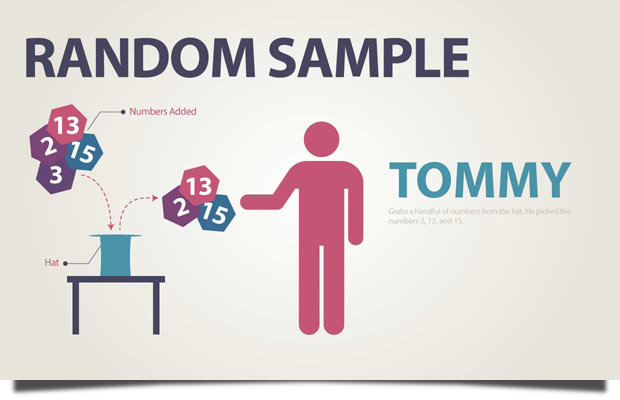# Random Sample

Random Sample is when members from the population are selected in such a way that each individual member has the same chance of being selected.

-from Elementary Statistics  Triola (2009).

# ExampleIn this example, members of a population are numbered and put into a hat. Tommy randomly picks several numbers from the hat. This is just one example of a random sample because the member of each population has an equal chance of being selected.

## Related Terms

Randomization

Simple Random Sample